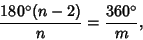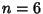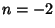## Crystallography Restriction

If a discrete Group of displacements in the plane has more than one center of rotation, then the only rotations that can occur are by 2, 3, 4, and 6. This can be shown as follows. It must be true that the sum of the interior angles divided by the number of sides is a divisor of 360°.whereis an Integer. Therefore, symmetry will be possible only forwhereis an Integer. This will hold for 1-, 2-, 3-, 4-, and 6-fold symmetry. That it does not hold foris seen by noting thatcorresponds to. Thecase requires that(impossible), and thecase requires that(also impossible).